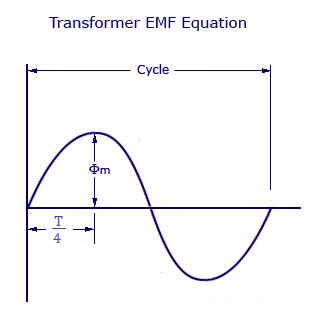# EMF Equation of Transformer and Voltage Transformation Ratio

0
512

### Transformer EMF Equation

#### In this article the amount of emf induced in the secondary winding due to the current supplied to the primary winding can be calculated by EMF equation of the transformer.This is very essential in the designing process of any transformer.Because the amount of emf induced due to mutual induction also depends on the number of turns in the coil.Transformer emf equation gives the output voltage value so that we can design a transformer as per our requirements.### Derivation : EMF Equation of Transformer

#### f = frequency of the AC supply (in Hz).### Voltage Transformation Ratio (K) of Transformer

3. #### Based on the requirement we can use any transformer as step-up or step down by changing the supply.

READ HERE  Parallel Operation of Transformers